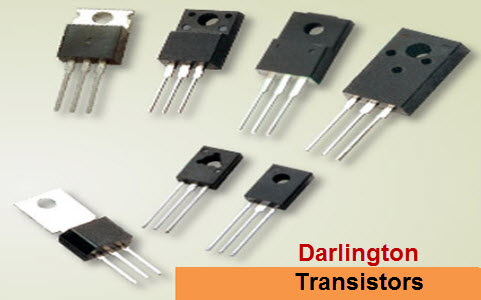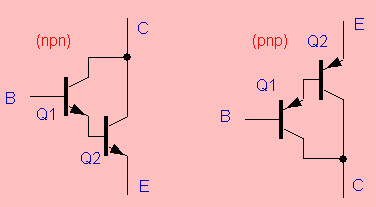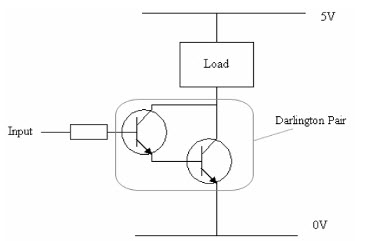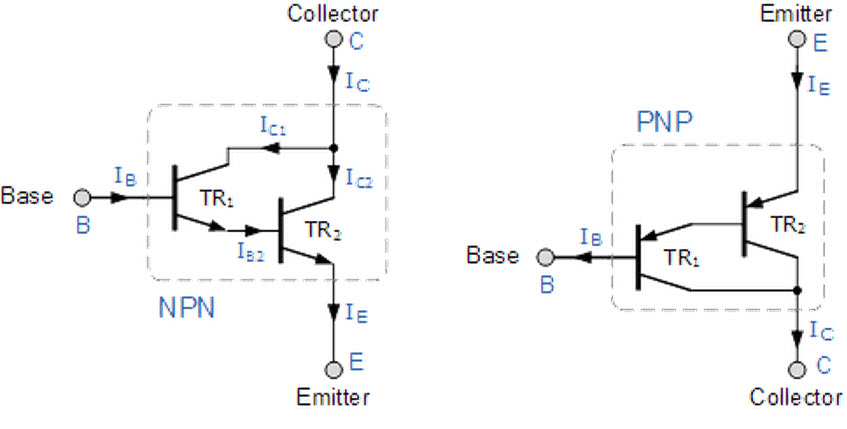# Darlington Transistor Working along with Applications

The term Darlington transistor is named from its inventor’s name Sidney Darlington. The Darlington transistor is made up of two PNP or NPN BJTs by connecting together. The emitter of the PNP transistor is connected to the base of the other PNP transistor to create a sensitive transistor with high current gain used in many applications where switching or amplification is crucial. The transistor pair in the Darlington transistor can be formed with two separately connected BJTs. As we know that, transistor is used as a switch as well as an amplifier, the BJT can be used to operate as an ON/OFF switch.Darlington TransistorDarlington Transistor

## Darlington Transistor

This transistor is also called as a Darlington pair, contains of two BJTs that are connected to deliver a high current gain from a low base current. In this transistor, the emitter of the i/p transistor is connected to the o/p of the base of the transistor and the collectors of the transistor are wired together. So, the i/p transistor amplifies the current even further amplifies by the o/p transistor. Darlington transistors are classified into different types by Power Dissipation, Max CE Voltage, Polarity, Min DC Current Gain and Type of Packaging. The common values of max CE voltage are 30V, 60V, 80V & 100V. The max CE voltage of Darlington transistor is 450V and power dissipation can be in the range of 200mW to 250mW.PNP and NPN Darlington Transistors

## Working of a Darlington Transistor

A Darlington transistor acts as a single transistor with high current gain, it means that a small amount of current is used from a microcontroller or a sensor to run a larger load. For instance, the following circuit is explained below. The below Darlington circuit is built with two transistors shown in the circuit diagram.Working of a Darlington Pair Transistor

### What is Current Gain?

Current gain is the most important characteristic of a transistor and it is indicated with hFE. When the Darlington transistor is switched ON, then the current supplies through the load to the circuit

Load current=i/p current X transistor gain

The current gain of every transistor varies. For a normal transistor the current gain would be normally around 100. So the current available to drive the load is 100 times greater than the i/p of the transistor.

The amount of i/p current to switch on a transistor is low in certain applications. So, a particular transistor cannot supply ample current to the load. So, the load current is equal to the i/p current and the gain of the transistor. If the input current increase is not possible, then the gain of the transistor will need to be increased. This process can be done by using a Darlington pair.

A Darlington transistor contains two transistors, but it acts as a single transistor with a current gain that equals. The total current gain is equal to current gains of the transistor1 and transistor 2.For instance, if you have two transistors with a similar current gain i.e 100

We know that, total current gain (hFE)= current gain of transisotr1 (hFE1) X current gain of transistor2 (hFE2)

100X100=10,000

You can observe in the above, it gives a vastly increased current gain compared with a single transistor. So, this will permit a low i/p current to switch a huge load current.

Generally, to turn on the transistor the base i/p voltage of the transistor must be greater (>) than 0.7 volts. In a Darlington transistor, two transistors are used. So the base voltage will be doubled 0.7×2=1.4V. When the Darlington transistor is turned On, then the voltage drop across the emitter & collector will be around 0.9V. So, if the supply voltage is 5V, the voltage across the load will be (5V – 0.9V = 4.1V)

### Structure of Darlington Transistor

The structure of Darlington transistor is shown below. For instance, here we have used NPN pair transistor. The collectors of the two transistors are connected together, and the emitter of the transistor TR1 energies the base terminal of the TR2 transistor. This structure attains β multiplication because for a base and collector current (ib and β. ib), where the current gain is greater than unity that is defined asStructure of Darlington Transistor

Ic=Ic1+Ic2
Ic= β1.IB+ β2.IB2

But the base current of the transistor TR1 is equal to IE1(emitter current), and emitter of the TR1 transistor is connected to the base terminal of the transistor TR2

IB2 = IE1
=Ic1+ IB
=β1.IB +IB
= IB(β1 + 1)

Substitute this IB2 value in the above equation

Ic= β1.IB+ β2. IB(β1 + 1)
IC= β1.IB + β2. IB β1 + β2. IB

= (β1+ (β2.β1) + β2). IB

In the above equation, β1 and β2 are gains of individual transistors.

Here, the overall current gain of the first transistor is multiplied by the second transistor that is specified by β, & a couple of bipolar transistors are combined to form a single Darlington transistor with a very high i/p resistance and value of β

#### Darlington Transistor Applications

This transistor is used in various applications where a high gain is required at a low frequency. Some applications are

• Power Regulators
• Audio Amplifier o/p stages
• Controlling of motors
• Display drivers
• Controlling of Solenoid
• Light and touch sensors.

This is all about Darlington transistor working with applications. We believe that you have got a better understanding of this concept. Furthermore, any queries regarding this topic or electronics projects, please give your feedback by commenting in the comment section below. Here is a question for you, what is the main function of a Darlington transistor?

Photo Credits: